We can use the law of equipartition of energy to determine specific heats of solids. Consider a solid of N atoms, each vibrating about its mean position. An oscillation in one dimension has average energy of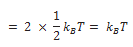In three dimensions, the average energy is 3kBT.

For a mole of solid, N = NA, and the total energy is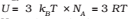Since for a solid ∆V is negligible, so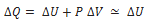Therefore,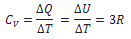## Specific Heat of water

We treat water like a solid. For each atom average energy is 3kBT. The water molecule has three atoms, two hydrogen and one oxygen. So it has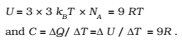This is the value observed and the agreement is very good. In the calorie, gram, degree units, water is defined to have unit specific heat. As 1 calorie = 4.179 joules and one mole of water is 18 grams, the heat capacity per mole is ~ 75 J mol-1 K-1 ~ 9R. However, with more complex molecules like alcohol or acetone the arguments, based on degrees of freedom, become more complicated.